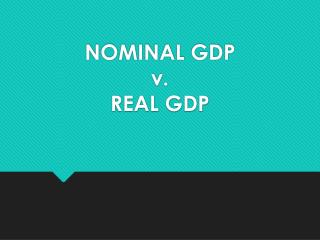DownloadDownload PresentationNOMINAL GDP v. REAL GDP

# NOMINAL GDP v. REAL GDP

Télécharger la présentation## NOMINAL GDP v. REAL GDP

- - - - - - - - - - - - - - - - - - - - - - - - - - - E N D - - - - - - - - - - - - - - - - - - - - - - - - - - -
##### Presentation Transcript

1. NOMINAL GDP v. REAL GDP

2. DEFINITIONS • Nominal GDP is the market value of all final goods and services produced in a given year. It is calculated as (Price X Quantity = Nominal GDP) • Real GDP is the inflation/deflation adjusted market value of all final goods and services produced in a given year relative to a base year. Real GDP represents the true purchasing power of our money. It describes how far our money will go. One way to calculate Real GDP is (base year price X current year quantity = Real GDP)

3. GDP PRICE INDEX • Definition: A price index is a measure of the price of a specified collection of goods and services, a “market basket” in a given year as compared to the price of an identical “market basket” of goods and services in a reference year. Price of market basket in Price Index specific year in = ----------------------------------- X 100 given year Price of the same market basket in the base year

4. GDP PRICE INDEX • The GDP Price Index allows accountants to make comparison between different years possible. • The GDP Price Index also allows accountants to adjust nominal GDP to real GDP for that year.

5. To calculate the price index for 2004 take the value of the market basket in 2004 (\$30) and divide it by the value of the market basket in 2001 (\$10) and multiply by 100. For 2005 divide 28 by 10 X 100

6. To find the Nominal GDP for 2004 multiply the units of output in 2004 (10) by the price of the market basket in 2004 (\$30). Determine Nominal GDP for 2005 in the same way.

7. Real GDP shows the market value of each year’s output measured in terms of dollars that have the same purchasing power as dollars had in the base year. One way to determine Real GDP is to divide nominal GDP by the price index. For 2005 divide 308 by 280 multiplied by 100

8. A second method to determine Real GDP is to multiply the output of a year in question by the market basket price in the base year. To determine Real GDP for 2002 multiply the output of 2002 (7) by the market basket price in the base year (\$10)

9. To determine the Price Index a different procedure would be to divide Nominal GDP by Real GDP times 100. For 2002 divide Nominal (\$140) by Real GDP (\$70) and multiply by 100.